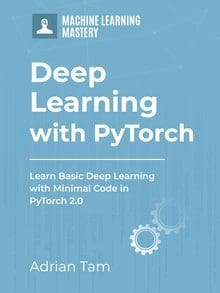# Introduction to Softmax Classifier in PyTorch

Last Updated on April 8, 2023

While a logistic regression classifier is used for binary class classification, softmax classifier is a supervised learning algorithm which is mostly used when multiple classes are involved.

Softmax classifier works by assigning a probability distribution to each class. The probability distribution of the class with the highest probability is normalized to 1, and all other probabilities are scaled accordingly.

Similarly, a softmax function transforms the output of neurons into a probability distribution over the classes. It has the following properties:

1. It is related to the logistic sigmoid, which is used in probabilistic modeling and has similar properties.
2. It takes values between 0 and 1, with 0 corresponding to an impossible event and 1 corresponding to an event that is certain to occur.
3. The derivative of softmax with respect to input x can be interpreted as predicting how likely it is that a particular class will be selected, given an input x.

In this tutorial, we’ll build a one-dimensional softmax classifier and explore its functionality. Particularly, we’ll learn:

• How you can use a Softmax classifier for multiclass classification.
• How to build and train a Softmax classifier in PyTorch.
• How to analyze the results of the model on test data.

Kick-start your project with my book Deep Learning with PyTorch. It provides self-study tutorials with working code.

Let’s get started.Introduction to Softmax Classifier in PyTorch.
Picture by Julia Caesar. Some rights reserved.

## Overview

This tutorial is in four parts; they are

• Preparing Dataset
• Build the Model with nn.Module
• Training the Classifier

## Preparing Dataset

First, let’s build our dataset class to generate some data samples. Unlike the previous experiments, you will generate data for multiple classes. Then you will train the softmax classifier on these data samples and later use it to make predictions on test data.

In below, we generate data for four classes based on a single input variable:

Let’s create the data object and check the first ten data samples and their labels.

This prints:

## Building the Softmax Model with nn.Module

You will employ nn.Module from PyTorch to build a custom softmax module. It is similar to the custom module you built in previous tutorials for logistic regression. So, what’s the difference here? Previously you used 1 in place of n_ouputs for binary classification, while here we’ll define four classes for multi-class classification. Secondly, in the forward() function, the model doesn’t use logistic function for prediction.

Now, let’s create the model object. It takes a one-dimensional vector as input and predicts for four different classes. Let’s also check how parameters are initialized.

This prints

### Want to Get Started With Deep Learning with PyTorch?

Take my free email crash course now (with sample code).

Click to sign-up and also get a free PDF Ebook version of the course.

## Training the Model

Combined with the stochastic gradient descent, you will use cross entropy loss for model training and set the learning rate at 0.01. You’ll load the data into the data loader and set the batch size to 2.

Now that everything is set, let’s train our model for 100 epochs.

After the training loop completed, you call the max() method on the model to make predictions. The argument 1 returns maximum value with respect to axis one, i.e., to return the index of the maximum value from each column.

From above, you should see:

These are the model predictions on test data.

Let’s also check the model accuracy.

In this case, you may see

Which in this simple model, you can see the accuracy approach 1 if you train it longer.

Putting everything together, the following is the complete code:

## Summary

In this tutorial, you learned how to build a simple one-dimensional softmax classifier. Particularly, you learned:

• How you can use a Softmax classifier for multiclass classification.
• How to build and train a Softmax classifier in PyTorch.
• How to analyze the results of the model on test data.

## Get Started on Deep Learning with PyTorch!#### Learn how to build deep learning models

...using the newly released PyTorch 2.0 library

Discover how in my new Ebook:
Deep Learning with PyTorch

It provides self-study tutorials with hundreds of working code to turn you from a novice to expert. It equips you with
tensor operation, training, evaluation, hyperparameter optimization, and much more...